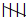Data Handling (EXERCISE – 9.1)EX 9.1 QUESTION 1.

In a Mathematics test, the following marks were obtained by 40 students. Arrange these marks in a table using tally marks.1. In a Mathematics test, the following marks were obtained by 40 students. Arrange these marks in a table using tally marks.

 8 1 3 7 6 5 5 4 4 2 4 9 5 3 7 1 6 5 2 7 7 3 8 4 2 8 9 5 8 6 7 4 5 6 9 6 4 4 6 6

(a) Find how many students obtained marks equal to or more than 7.

(b) How many students obtained marks below 4?

Solution:

 Marks Tally Marks Number of Students 1 || 2 2 ||| 3 3 ||| 3 4|| 7 5| 6 6|| 7 75 8 |||| 4 9 ||| 3

(a) Number of students who obtained marks equal to or more than 7

= 5 + 4 + 3 = 12

(b) Number of students who obtained marks below 4

= 2 + 3 + 3 = 8.

EX 9.1 QUESTION 2.

Following is the choice of sweets of 30 students of Class VI.

(a) Arrange the names of sweets in a table using tally marks.

(b) Which sweet is preferred by most of the students?

Solution:

 Sweets Tally Marks Number of Students Ladoo| 11 Barfi ||| 3 Jalebi|| 7 Rasgulla|||| 9 Total 30

(b) Ladoo is preferred by most of the students.

EX 9.1 QUESTION 3.

Catherine threw a dice 40 times and noted the number appearing each time as shown below :

 1 3 5 6 6 3 5 4 1 6 2 5 3 4 6 1 5 5 6 1 1 2 2 3 5 2 4 5 5 6 5 1 6 2 3 5 2 4 1 5

Make a table and enter the data using tally marks. Find the number that appeared.

(a) The minimum number of times

(b) The maximum number of times

(c) Find those numbers that appear an equal number of times.

Solution:

 Numbers Tally Marks Number of times 1|| 7 2| 6 35 4 |||| 4 5| 11 6|| 7

The number that appeared-

(a) The minimum number of times = 4

(b) The maximum number of times = 5

(c) Those numbers that appear an equal number of times = 1 and 6

EX 9.1 QUESTION 4.

Following pictograph shows the number of tractors in five villages.(a) The village has the minimum number of tractors = D  i.e., 3.

(b) The village has the maximum number of tractors = C  i.e., 8.

(c) Village B has 5 tractors

Village C has 8 tractors

= 8 – 5

= 3 tractors

(d) Total number of tractors in all the villages = 6 + 5 + 8 + 3 + 6 = 28 tractors

EX 9.1 QUESTION 5.

The number of girl students in each class of a co-educational middle school is depicted by the pictograph:(a) Class VIII has the minimum number of girl students=  1x 4 = 6.
(b) No, number of girls in Class VI = 4 x 4 = 16

and number of girls in Class V = 2$\frac { 1 }{ 2 }$ x 4 = 10

no, the number of girl students in Class VI is not less than that of Class V.
(c) Number of girls in Class VII = 3 x 4 = 12

EX 9.1 QUESTION 6.

The sale of electric bulbs on different days of a week is shown below:(a) Bulbs sold on Friday = 7 x 2 = 14
(b) On Sunday, the number of bulbs sold = 9 x 2 = 18
(c) On Wednesday and Saturday, the same number of bulbs were sold= 4 x 2 = 8
(d) The minimum number of bulbs were sold on Wednesday and Saturday= 4 x 2 = 8
(e) Total number of bulbs sold in a week = 43
Number of cartons needed 5
= (43 x 2) ÷ 9 = 86 ÷ 9$\frac { 5 }{ 9 }$= 9 = 10 cartons.

EX 9.1 QUESTION 7.

In a village six fruit merchants sold the following number of fruit baskets in a particular season:(a) Martin sold the maximum number of fruit baskets=  9$\frac { 1 }{ 2 }$x 100 = 950
(c) Anwar sold =  7 x 100 = 700.
(c) Anwar, Martin, and Ranjit Singh have sold 600 or more fruit baskets and planning to buy a godown for the next season.